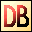DelphiBasicsInfinityConstant Floating point value of infinite size Math unit
 const Infinity =  1.0 / 0.0;
Description
The Infinity constant is a special floating point number, marked as infinite in size.

It may be assigned and calculated upon, but use IsInfinite for comparisons.
Related commands
 IsInfinite Checks whether a floating point number is infinite IsNaN Checks to see if a floating point number holds a real number NaN Not a real numberDownload this web site as a Windows program.